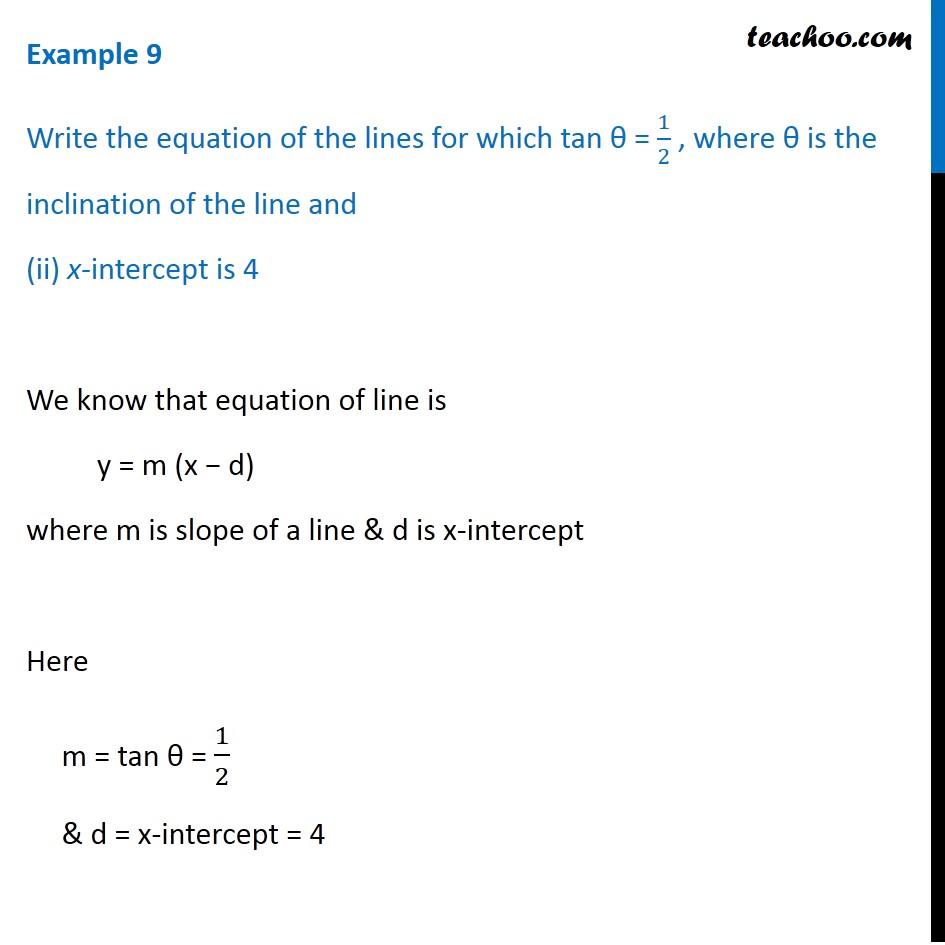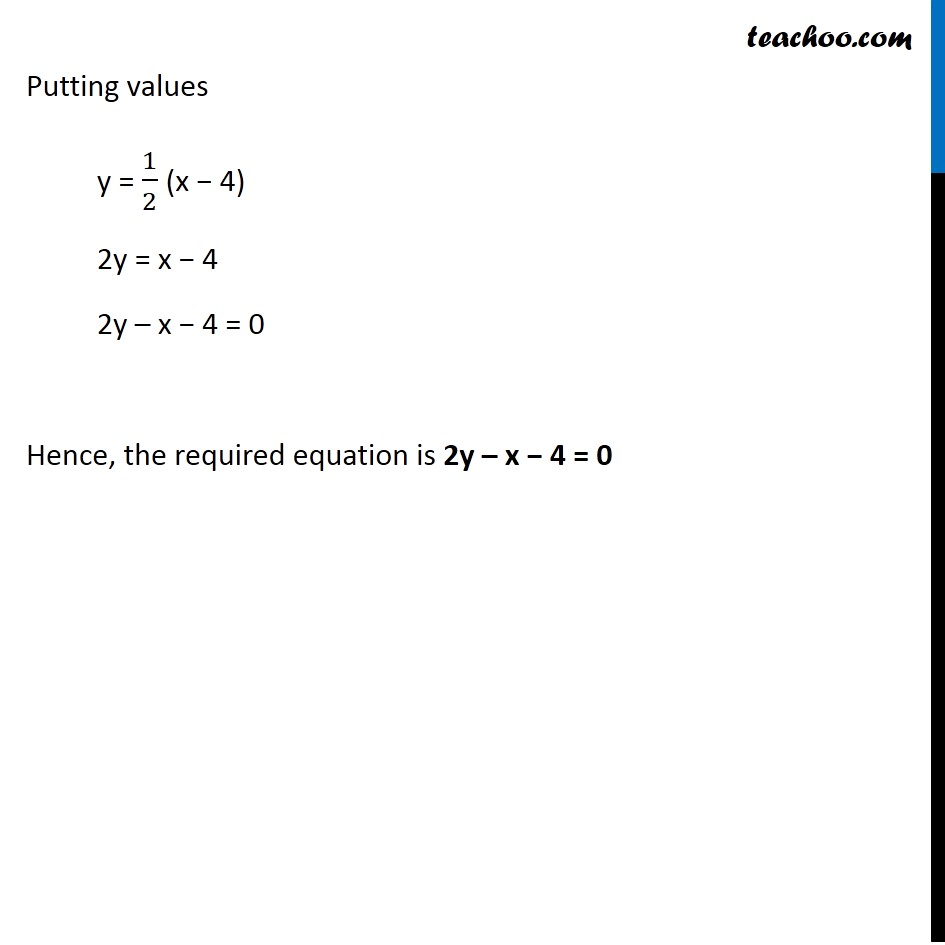Examples

Chapter 9 Class 11 Straight Lines
Serial order wiseLearn in your speed, with individual attention - Teachoo Maths 1-on-1 Class

### Transcript

Example 9 Write the equation of the lines for which tan θ = 1/2 , where θ is the inclination of the line and (ii) x-intercept is 4 We know that equation of line is y = m (x − d) where m is slope of a line & d is x-intercept Here m = tan θ = 1/2 & d = x-intercept = 4 Putting values y = 1/2 (x − 4) 2y = x − 4 2y – x − 4 = 0 Hence, the required equation is 2y – x − 4 = 0